TitleCollege Algebra
Tutorial 44: Logarithmic
PropertiesAnswer/Discussion to 1a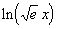What is the base in this problem? This time the base is e.  Make sure that you keep that same base throughout the problem.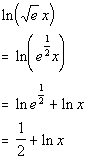*Use the product rule *Use the definition of logs to simplify *1/2 is the exponent needed on e to get e^1/2Answer/Discussion to 1b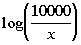What is the base in this problem? This time the base is 10.  Make sure that you keep that same base throughout the problem.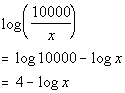*Use the quotient rule *Use the definition of logs to simplify *4 is the exponent needed on 10 to get 10000Answer/Discussion to 1c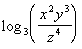What is the base in this problem? This time the base is 3.  Make sure that you keep that same base throughout the problem.*Use the product rule and the quotient rule *Use the power ruleAnswer/Discussion to 2a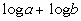What is the base in this problem? This time the base is 10.  Make sure that you keep that same base throughout the problem.*Use the product ruleAnswer/Discussion to 2b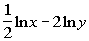What is the base in this problem? This time the base is e.  Make sure that you keep that same base throughout the problem.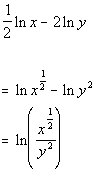*Use the power rule *Use the  quotient ruleAnswer/Discussion to 3a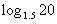Note that the calculator can only evaluate logs that are base 10 or base e.  Since this problem is in base 1.5,  we need to change the base to base e (or base 10).  Since the instructions say base e, let's do that.*Use the calculator to find ln 20 and ln 1.5   *Divide

Last revised on March 23, 2011 by Kim Seward.